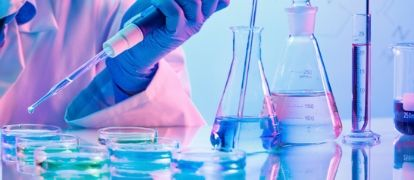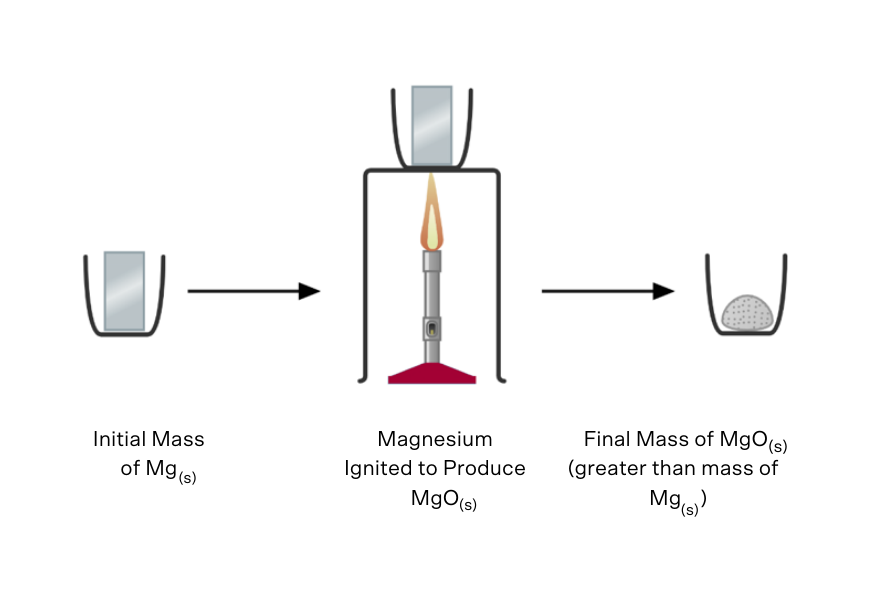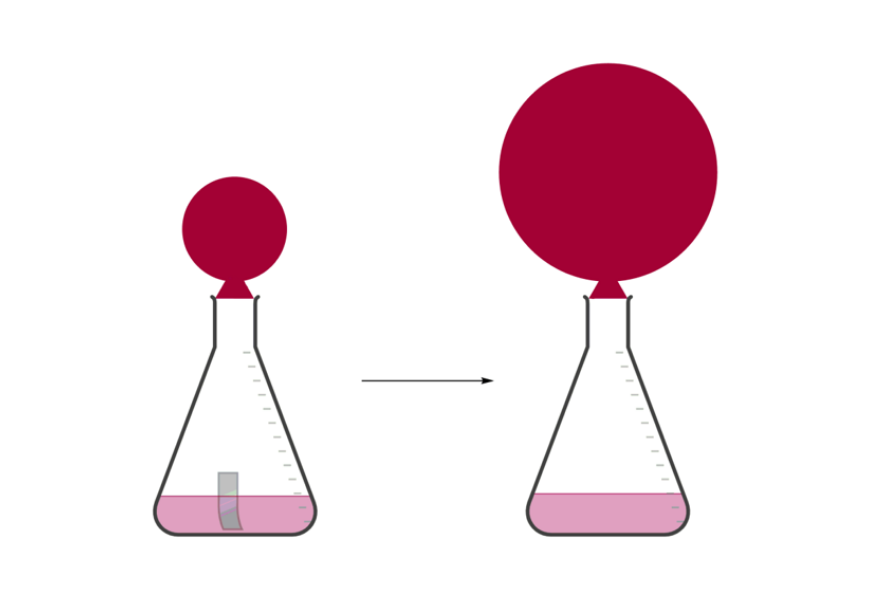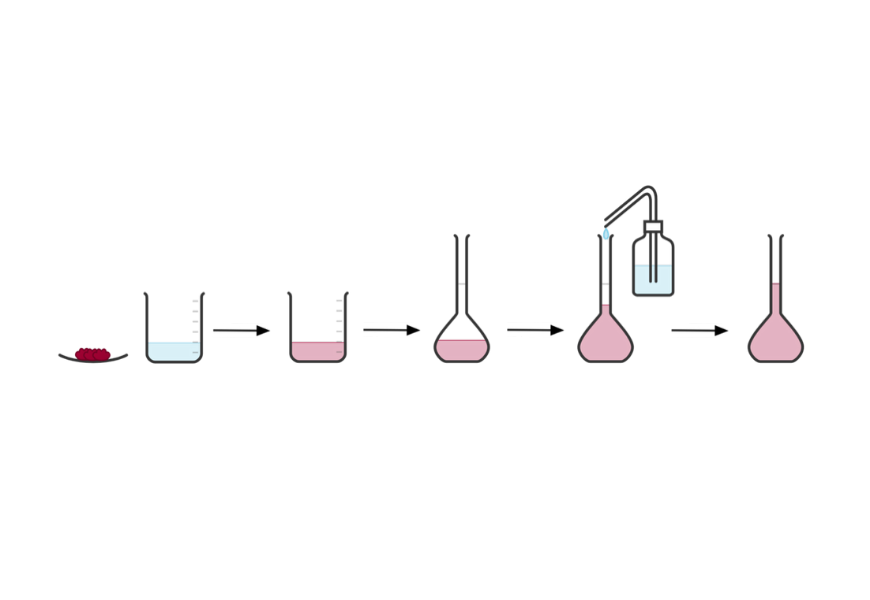# Part 2: Introduction To Quantitative Chemistry

Do you struggle with Quantitative Chemistry in Year 11? In this article, we provide you with an introduction to Quantitative Chemistry and the fundamental ideas you need to know including the mole theory and stoichiometry.In this article, we provide you with an introduction to Quantitative Chemistry. Quantitative Chemistry is the branch of Chemistry that allows chemists to apply maths to chemical equations to work out the amount of different substances present.

To begin with, we will look at the fundamental ideas you need including the mole theory and stoichiometry.A free pocket-sized cheatsheet, so you're always prepared.

## Chemical Reactions and Stoichiometry

Quantitative Chemistry is about calculating the amounts of materials in a substance or the quantity of something that could be made from a particular material.

Practical investigations are a means of you learning how to learn and develop these skills.

### Practical investigations

Practical investigations are an essential part of chemistry, and involve changes in the quantity of a chemical present.

You are expected to be able to propose methods to measure changes in the quantity of a substance that might occur during a chemical reaction. You could be expected to measure the change in the mass of a substance as a result of a chemical reaction.

For example, if a strip of magnesium ribbon was burnt in oxygen to form magnesium oxide, you could measure the change in mass of the solid substance, and work out what mass of oxygen was added to the material.You could also be expected to measure volumes of gas produced in the reaction using things like a syringe or a balloon. For example, hydrogen is produced when metals react with acid, and can be captured using a balloon.When asked to propose how an experiment to measure mass or volume could be done, remember to think in practical terms: choose a method that is straightforward and achievable in a basic laboratory.

## Stoichiometry and the Law of Conservation of Mass

The Law of Conservation  of Mass states that mass cannot be created or destroyed by chemical reactions or physical transformations. This means that the total mass of the products in a chemical reaction are exactly the same as the total mass of the reactants – matter cannot be produced from nothing!

A consequence of the Law of Conservation of Mass is that all chemical equations must be balanced, that is, you must show the same number of each type of atom on each side of the reaction arrow. In order for a chemical equation to be balanced and correct:

• All reactants and products must be included
• The chemical formula for each chemical species must be correct
• The physical state at room temperature and pressure must be included
• The number of each type of atom must be the same on each side of the reaction arrow.

For example, the balanced chemical equation for the reaction between magnesium metal and oxygen gas to produced magnesium oxide is as follows: $$2Mg_{(s)} + O_{2(g)} \rightarrow 2MgO_{(g)}$$.

As an additional note, you need to know the following polyatomic ions. If you don’t, learn them now!

 Polyatomic Ion Formula Ammonium $$NH_4^{ +}$$ Hydroxide $$OH^-$$ Nitrate $$NO_3^-$$ Sulfate $$SO_4^{ 2-}$$ Carbonate $$CO_3^{2-}$$ Phosphate $$PO_4^{3-}$$

To measure the quantities of different chemicals, we need a unit – the mole. It’s exactly the same as measuring an amount of eggs in dozens, or the amount of time that has passed in hours.

Let’s take a look at how the mole concept is applied.

## The Mole Concept

The mole concept will be crucial in all of the chemistry you encounter from this point onward. The mole is defined as $$6.022 \times 10^{23}$$ atoms. This very large number is referred to as Avogadro’s number $$N_A$$.

In a chemical reaction, different compounds will react in simple whole number ratios by moles. This is known as the stoichiometric ratio, or mole ratio in a chemical reaction. An example of this is shown below, when hydrogen and oxygen combine to form water.

$$2H_{2(g)} + O_{2(g)}→ 2H_{2}O_{(l)}$$

We would say that the above reaction has a $$2:1:2$$ mole ratio. You should be able to find the mole ratio for any chemical reaction.

A related concept is the molar mass of a substance $$MM$$ which is the mass in grams of one mole of that substance.

You are required to know how to perform a lot of calculations involving moles. These include:

• The number of moles $$n$$ of a mass $$m$$, which is given by $$n = \frac{m}{MM}$$
• The number of particles $$p$$ in a given number of moles, which is given by $$p=n \times N_A$$
• The percentage composition of an element in a compound.
• The empirical formula of a compound, which is the simplest mole ratio between different elements in that compound.
• Limiting reagent calculations, where you have different amounts of two or more reactants. The reactant that limits the formation of products (because it runs out first) is the limiting reagent.

## Concentration and Molarity

Once we know the quantity of a substance (in moles), we can calculate the concentration of that substance, usually in moles per litre. This is very useful for when reactants are dissolved in water, and we need to quantify the amount taking part in a chemical reaction.

To calculate concentration of a given substance in solution, the molarity formula is used.

 $$c=\frac{n}{V}$$ $$c$$ = concentration ($$\text{molL}^{-1}$$), $$n$$ = number of moles of solute, $$V$$ = volume of solution($$\text{L}$$)

You also need to know how to make a standard solution. A standard solution is a solution of known concentration. It is normally prepared from a primary standard. An exact weight of a solid is measured to give the number of moles required for a given concentration of solution. The exact volume of solution is generated using a volumetric flask.

You will also be expected to perform dilution calculations. To do this, the number of moles in a given solution is calculated. The number of moles in the solution will not change. After some solvent is added or removed, the new volume is calculated, and the final concentration of the solution can be determined using the dilution formula: $$c_1V_1= c_2 V_2$$.We’ve discussed solids and liquids, but what happens if we are dealing with gases?

It turns out that gases have special properties that we can apply different laws to.

## Gas Law

It is also possible to relate the volume of a gas to the number of moles, but the volume of a gas will vary with temperature and pressure as described by the ideal gas law:

$$PV= nRT$$

Where:

• $$P$$ = the pressure ($$\text{kPa}$$),
• $$V$$ = the volume ($$\text{L}$$),
• $$n$$ = the number of moles,
• $$R$$ = the ideal gas constant ($$8.314 \text{ Jmol}^{-1}\text{K}^{-1}$$)
• $$T$$ = the temperature ($$\text{K}$$)

A number of older gas laws are closely related to the ideal gas law, however they can only be used when some property or combinations of properties of the gas change. These are summarised in the table below.

 Law Equation What changes What stays constant Gay-Lussac’s Law $$\frac{P_1}{T_1} = \frac{P_2}{T_2}$$ Pressure, temperature Volume, number of moles Boyle’s Law $$P_1 V_1= P_2 V_2$$ Pressure, volume Temperature, number of moles Charles’ Law $$\frac{V_1}{T_1} = \frac{V_2}{T_2}$$ Volume, temperature Pressure, number of moles Avogadro’s Law $$\frac{V_1}{n_1} = \frac{V_2}{n_2}$$ Volume, number of moles Pressure, temperature

## Want to improve your calculation skills?

© Matrix Education and www.matrix.edu.au, 2022. Unauthorised use and/or duplication of this material without express and written permission from this site’s author and/or owner is strictly prohibited. Excerpts and links may be used, provided that full and clear credit is given to Matrix Education and www.matrix.edu.au with appropriate and specific direction to the original content.